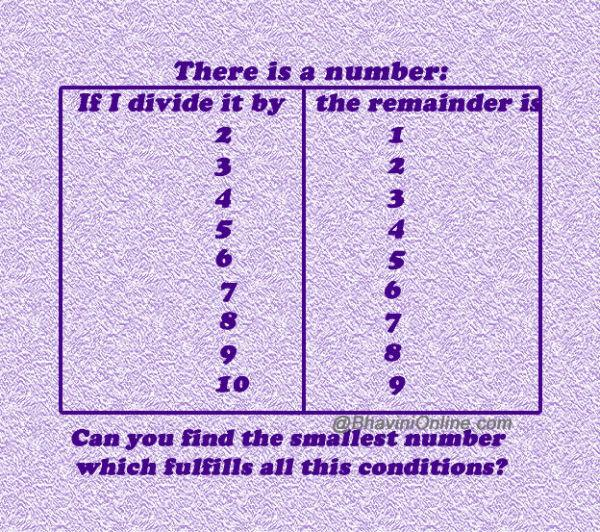# Find the Number From The Given Conditions

Have fun with numbers with this mathematical riddle.

There is a number:

If I divide it by 2, the remainder is 1.

If I divide it by 3, the remainder is 2.

If I divide it by 4, the remainder is 3.

If I divide it by 5, the remainder is 4.

If I divide it by 6, the remainder is 5.

If I divide it by 7, the remainder is 6.

If I divide it by 8, the remainder is 7.

If I divide it by 9, the remainder is 8.

If I divide it by 10, the remainder is 9.

There is a number:

 If I divide it by the remainder is 2 1 3 2 4 3 5 4 6 5 7 6 8 7 9 8 10 9

Can you find the smallest number which fulfills all this conditions?So were you able to solve the riddle? Leave your answers in the comment section below.